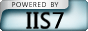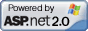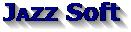Contact us?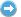JazzSoft@live.com     English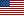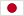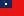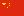Factory Automation & Yield Management Solution Provider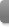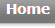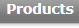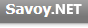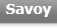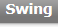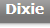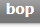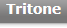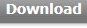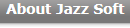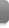Skip Navigation LinksHome > Products > Swing > Swing SECS II Reference > Properties > Pointer

Swing SECS II Reference

Pointer Property

The node used as candidate for operation. Node consists of '/' (slash), node number, and '[]' (bracket). Node number is the number which begins from 1. It is considered that root was specified when node is empty. Node can be compared to the directory structure of MS-DOS (I think it may be easy to understand that the description of 'node' in explanation is replaced with a 'directory').

To create node item, first specify node then add command to it.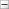Syntax

 Visual Basic

 Visual C++Return ValueExample

 Visual Basic .Pointer = "1" .Add SecsTypeAscii, "abc"

 Visual C++ m_msg.SetPointer("1"); m_msg.Add(SecsTypeAscii,"abc");Remarks

You can put 'slush' in front of the node string. It will be ignored.

 Visual Basic .Pointer = "" .Pointer = "/"      ' This is the same .Pointer = "1/2" .Pointer = "/1/2"   ' This is also the same

 Visual C++ m_msg.SetPointer(""); m_msg.SetPointer("/");      // This is the same m_msg.SetPointer("1/2"); m_msg.SetPointer("/1/2");   // This is also the same

Node can be made under the other node. In this case, you have to make parent node ahead of child node. The order to make nodes may differ from the order of actual SECS-II buffer.

1 : {
2 :   {
3 :     <a'abc'>
4 :   }
5 :   {
6 :     <a'def'>
7 :   }
8 : }

The order to add nodes are no matter and flexible whether "1, 2, 3, 5 then 6", "1, 2, 5, 3 then 6" or "1, 2, 5, 6 then 3".

1, 2, 3, 5 then 6

 Visual Basic .Pointer = "" .Add SecsTypeList, ""       ' 1 .Pointer = "1" .Add SecsTypeList, ""       ' 2 .Pointer = "1/1" .Add SecsTypeAscii, "abc"   ' 3 .Pointer = "2" .Add SecsTypeList, ""       ' 5 .Pointer = "2/1" .Add SecsTypeAscii, "def"   ' 6

 Visual C++ m_msg.SetPointer(""); m_msg.Add(SecsTypeList,"");       // 1 m_msg.SetPointer("1"); m_msg.Add(SecsTypeList,"");       // 2 m_msg.SetPointer("1/1"); m_msg.Add(SecsTypeAscii,"abc");   // 3 m_msg.SetPointer("2"); m_msg.Add(SecsTypeList,"");       // 5 m_msg.SetPointer("2/1"); m_msg.Add(SecsTypeAscii,"def");   // 6

1, 2, 5, 3, then 6

 Visual Basic .Pointer = "" .Add SecsTypeList, ""       ' 1 .Pointer = "1" .Add SecsTypeList, ""       ' 2 .Pointer = "2" .Add SecsTypeList, ""       ' 5 .Pointer = "1/1" .Add SecsTypeAscii, "abc"   ' 3 .Pointer = "2/1" .Add SecsTypeAscii, "def"   ' 6

 Visual C++ m_msg.SetPointer(""); m_msg.Add(SecsTypeList,"");       // 1 m_msg.SetPointer("1"); m_msg.Add(SecsTypeList,"");       // 2 m_msg.SetPointer("2"); m_msg.Add(SecsTypeList,"");       // 5 m_msg.SetPointer("1/1"); m_msg.Add(SecsTypeAscii,"abc");   // 3 m_msg.SetPointer("2/1"); m_msg.Add(SecsTypeAscii,"def");   // 6

1, 2, 5, 6 then 3

 Visual Basic .Pointer = "" .Add SecsTypeList, ""       ' 1 .Pointer = "1" .Add SecsTypeList, ""       ' 2 .Pointer = "2" .Add SecsTypeList, ""       ' 5 .Pointer = "2/1" .Add SecsTypeAscii, "def"   ' 6 .Pointer = "1/1" .Add SecsTypeAscii, "abc"   ' 3

 Visual C++ m_msg.SetPointer(""); m_msg.Add(SecsTypeList,"");       // 1 m_msg.SetPointer("1"); m_msg.Add(SecsTypeList,"");       // 2 m_msg.SetPointer("2"); m_msg.Add(SecsTypeList,"");       // 5 m_msg.SetPointer("2/1"); m_msg.Add(SecsTypeAscii,"def");   // 6 m_msg.SetPointer("1/1"); m_msg.Add(SecsTypeAscii,"abc");   // 3

When you read node value, first specify node then read.

 Visual Basic .Pointer = "1" If .Value = "abc" Then   ... End If

 Visual C++ m_msg.SetPointer("1"); if(m_msg.GetValue()=="abc") {   ... }

If node is array, they are returned separated with space code.

 Visual Basic .Pointer = "1" .Add SecsTypeBool, "1" .Add SecsTypeBool, "0" ... .Pointer = "1" Dim strBuf As String strBuf = .Value   ' "1 0" is returned

 Visual C++ m_msg.SetPointer("1"); m_msg.Add(SecsTypeBool,"1"); m_msg.Add(SecsTypeBool,"0"); ... m_msg.SetPointer("1"); CString strBuf=m_msg.GetValue();   // "1 0" is returned

If you extract one of the item in array, use bracket. Index of array begins with 0.

 Visual Basic .Pointer = "1" Dim strBuf As String strBuf = .Value   ' "0" is returned

 Visual C++ m_msg.SetPointer("1"); CString strBuf=m_msg.GetValue();   // "0" is returned

So if root node is array, it becomes strange format -- only bracket.

 Visual Basic .List = "" .Pointer = "" Dim strBuf As String strBuf = .Value   ' "3.14" is returned

 Visual C++ m_msg.SetList(""); m_msg.SetPointer(""); Cstring strBuf=m_msg.GetValue();   // "3.14" is returned

Bracket is ignored in ASCII, JIS-8 and List.See Also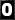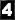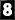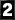Contact us?JazzSoft@live.com Copyright © 1997 - 2019 Jazz Soft, Inc.